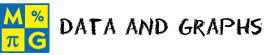Data and GraphsThis page lists the Learning Objectives for all Data and Graphs lessons in Unit 11.

Data and Line Graphs

The student will be able to:

• Define data, line graph, title, label, scale, point and line.
• Identify the parts of a line graph.
• Examine line graphs presented in examples.
• Interpret information from line graphs presented in five interactive exercises.

Constructing Line Graphs

The student will be able to:

• Define range, horizontal scale and vertical scale.
• Examine the procedure for constructing a line graph from a given set of data.
• Describe the procedure for constructing a line graph.
• Construct line graphs for data presented in two exercises.

Data and Bar Graphs

The student will be able to:

• Define bar graph, grid line and categories.
• Identify the parts of a bar graph.
• Examine bar graphs presented in examples.
• Recognize that bar graphs can have vertical or horizontal scales.
• Interpret information from bar graphs presented in five interactive exercises.

Constructing Bar Graphs

The student will be able to:

• Define bar, bar length and bar height.
• Examine the procedure for constructing a bar graph from a given set of data.
• Describe the procedure for constructing a bar graph.
• Construct bar graphs for data presented in two exercises.

Data and Circle Graphs

The student will be able to:

• Define circle graph, sector and pie chart.
• Identify the parts of a circle graph.
• Examine circle graphs presented in examples.
• Recognize that a circle graph is easier to read when percents, not fractions, are used to label the data
• Explain why a circle graph is also called a pie chart.
• Recognize, if there are more than five or six categories in a set of data, then a circle graph is not a good choice for displaying that data.
• Recognize that circle graphs provide a visual presentation of the whole and its parts.
• Interpret information from circle graphs presented in five interactive exercises.

Constructing Circle Graphs

The student will be able to:

• Review the definition and purpose of a circle graphs.
• Examine the procedure for constructing a circle graph from a given set of data presented in table.
• Compute the values for the parts and the whole of the graph.
• Compute exactly what fraction or percent each item (part) represents.
• Compute the angle measure for each part of a circle graph.
• Draw and label the parts of a circle graph.
• Describe the procedure for constructing a circle graph.
• Recognize that circle graphs represent data visually in the same proportion as the numerical data given in a table.
• Construct circle graphs for data presented in two exercises.

Comparing Graphs

The student will be able to:

• Define visual data display.
• Describe the types of graphs learned in this unit and their uses.
• Compare and contrast different graphs.
• Recognize that circle graphs are best used to compare the parts of a whole.
• Recognize that bar graphs are used to compare facts.
• Recognize that a line graph is used to show changes over time.
• Determine which type of graph is appropriate for representing a given set of data presented in five interactive exercises.
• Connect graphs to data found in the real world.

Practice Exercises

The student will be able to:

• Examine ten interactive exercises for all topics in this unit.
• Interpret and analyze graphs presented to determine what information is given.
• Complete exercises by applying data and graph concepts.
• Self-assess knowledge and skills acquired from this unit.

Challenge Exercises

The student will be able to:

• Evaluate ten interactive exercises for all topics in this unit.
• Interpret and analyze graphs presented to determine what information is given.
• Compare a set of graphs that represent the same data in each of three exercises.
• Determine which graph shows all of the facts correctly.
• Determine which type of graph is appropriate for representing a given set of data in each of three exercises.
• Determine what is wrong with graphs in each of four exercises.
• Develop problem-solving skills.

Solutions

The student will be able to:

• Examine the answer for each exercise presented in this unit.
• Examine graphs presented as possible solutions..
• Recognize that graphs created will vary depending on the scales used.
• Compare possible solutions to completed exercises
• Identify and evaluate incorrect answers.
• Reconstruct and label graphs that did not accurately represent the data from completed exercises.
• Identify areas of strength and weakness.
• Decide which concepts and procedures need to be reviewed from this unit.